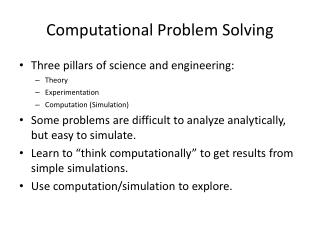DownloadDownload PresentationComputational Problem Solving

Computational Problem Solving

Télécharger la présentationComputational Problem Solving

- - - - - - - - - - - - - - - - - - - - - - - - - - - E N D - - - - - - - - - - - - - - - - - - - - - - - - - - -
Presentation Transcript

1. Computational Problem Solving • Three pillars of science and engineering: • Theory • Experimentation • Computation (Simulation) • Some problems are difficult to analyze analytically, but easy to simulate. • Learn to “think computationally” to get results from simple simulations. • Use computation/simulation to explore.

2. Computational Example 1 • Birthday problem: Among a group of n people, what is the probability that two share a birthday? • This is related to hashing. • Can you determine this analytically? • How can you do this with simulation?

3. Algorithm #1 bool birthday(int count) { int myArray; for (int i=0; i<count; i++) { int pos = Random(365); if (myArray[pos] != 0) return true; else myArray[pos] = 1; } return false; } Issue: Must do it enough times to get meaningful statistics

4. Algorithm #2 double birthday(int count, int numtrials) { int myArray; int hits = 0; for (int trial=0; trial<numtrials; trial++) { for (int i=0; i<365; i++) myArray[i] = 0; for (int i=0; i<count; i++) { int pos = Random(365); if (myArray[pos] != 0) { hits++; break; } else myArray[pos] = 1; } } return (double)hits/(double)numtrials; }

5. Computational Problem 2 • Analysis of hashing: What should we expect from a good hash function in terms of number of slots hit, length of chains? • Possible to analyze “ideal” performance analytically, but harder than simulating • Very hard or impossible to analyze performance of real hash functions analytically, but easy with simulation.

6. Things to Know • Performance Measures: • How many slots were used (average)? • What is the minimum for slots used? • What is the longest chain ever? • What is the average for longest chain? • What is the expected cost? • Issues: • Data Distribution • Fill factor • Table size

7. Computational Example 3 • Do you know an algorithm to compute a square root? • Assuming that you know how to multiply, can you think of a way to compute square roots? • Guess/convergence testing is a fundamental concept for many numerical methods.

8. Algorithm double squareRoot(double val) { double lower, upper; upper = val; if (val < 1) lower = 0; else lower = 1; while ((upper – lower) > EPSILON) { double curr = (upper + lower)/2.0; if ((curr * curr) > val) upper = curr; else lower = curr; } }

9. Computational Example 4 • Problem: design a traffic light for an intersection • Must allow every traffic direction access to the intersection in a reasonable length of time • Goal: maximize the total traffic flow possible through the intersection • Other goals are possible • Part of solution: traffic simulation

10. Traffic Simulation • Consider all car directions, both from and to • Traffic arrives at random, but typical, intervals • Traffic light has a small number of states and timers • State transitions are programmed in light • Simulation program runs simulated traffic through the intersection and measures the worst-case behavior • Vary the state transitions to investigate different design possibilities